Alternators Connected to Infinite Bus bar

In the previous post we have seen that synchronous generators do not operate individually in a generating station but they are interconnected so that total generating capacity will be high.When number of alternators are interconnected forming a system which may be treated as an infinite bus. Infinite bus bar is one which keeps constant voltage and frequency although the load varies. Thus it may behave like a voltage source with zero internal impedance and infinite rotational inertia. Any alternator switched on to or off, the infinite bus does not cause any change in the voltage and frequency of the system.
The characteristics of a synchronous generator on infinite bus bars are quite different from those when it is connected to another alternator and both are in parallel. When two alternators are connected in parallel we have seen that a change in the excitation changes the terminal voltage and p.f. is determined by load. However change in excitation for an alternator connected to infinite bus bar will not change the terminal voltage but the power factor only is affected whereas the power developed by an alternator depends only on mechanical power input.
Now we will consider the effect of excitation and driving torque on the performance of an alternator which is connected to infinite bus bar. In all the further discussion we will take zero losses for the machine.
1.1 Effect of Excitation
Let us consider the cylindrical rotor as shown in the Fig.1 connected to infinite busbar.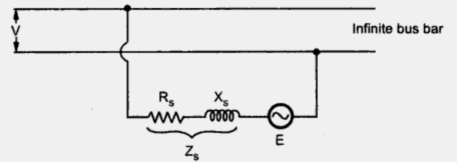Fig. 1
The voltage equation can be written as
where     E = Induced e.m.f. or excitation e.m.f.
V = Constant bus voltage
I = Armature current
Z= Synchronous impedance
The same equation neglecting the armature resistance can be rewritten as,
Again we will consider the two cases one with alternator on no load and other with alternator on load.
1.1.1 Alternator on No Load
Since we are considering the losses to be zero the power angle will be zero. Thus the power transferred from or to the bus is zero ( P = ((EV/Xs) sinδ )
Now if the excitation is properly adjusted at no load then induced e.m.f. E will be equal to bus voltage V and no current will flow. This is shown in Fig. 2. This is floating condition of alterntor.Fig. 2                                                             Fig.3
Now if the alternator is under excited then induced e.m.f. E will be less that V. This will cause circulating current ISY to flow which will lead E by angle of 90o. Due to this it produces magnetizing m.m.f. which will try to increase field m.m.f. to maintain alternator terminal voltage equal to the bus bar voltage. This is shown in the following Fig. 3.
Similarly if alternator is over excited then induced e.m.f. will be more than V which will again cause a circulating current to ISY flow. The power angle δ is zero. This current lags E by 90o. This will produce demagnetizing armature m.m.f. which will counterbalance the effect of increased field m.m.f. and again the terminal voltage of an alternator will be equal to constant bus bar voltage V. This is represented in Fig. 4.Fig. 4
It can be seen that in both the cases considered above, no active power is delivered since ISY is in quadrature with V and load angle is also zero. But alternator takes reactive power from bus since E < V and delivers it to bus if E > V.
1.1.2 Alternator on Load
Now let us consider that alternator is supplying power to an infinite bus which has induced e.m.f. E, power angle δ and working at unity power factor with current I.
With mechanical power input to the alternator remaining constant, the power given by (EV/Xs) sin δ will remain constant. If by varying excitation induced e.m.f. E is increased to E1 then the load angle will also change from   δ to   δ1. From the phasor diagram it can be determined as E1 sinδ1 = E sinδ as V and Xs are constant. The drop due to synchronous reactance also increases and armature current increases from I to I1. This current has two components one real component and other quadrature component. This quadrature component is nothing but demagnetizing component. This will result in lagging power factor cosΦ1.
Similarly if the excitation is decreased so that induced e.m.f. reduces from E to E2 with corresponding change in power angle from δ to  δ2. The armature current in this case will be I2 which has real component and magnetizing component which results in leading power factor cosΦ2. This can be represented in the phasor diagram shown in Fig. 5.Fig. 5
From the phasor diagram it can be seen that
I1 cosΦ1 = I2 cosΦ2 = 1
Multiplying by V throughout,
V I1 cosΦ1 = V I2 cosΦ2 = VI
This indicates that power delivered to the bus will remain constant. Thus by changing the field excitation the active power is unaltered. But change in excitation results in corresponding operating power factor as shown in phasor diagram.
Note : An under excited alternator operates at leading power factor whereas an over excited alternator operates at lagging power factor.
It can also be seen that armature current is minimum at unity power factor. For over excited alternator as E1 cos δ >V therefore as seen from case (i) i.e. no load condition alternator delivers reactive power to the bus whereas for underexcited alternator E2 cosδ < V, alternators takes reactive power from the bus. This variation of excitation and armature current can be plotted as shown in Fig. 6. This is known as curves for synchronous generators by virture of their shape.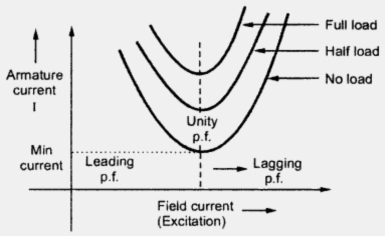Fig. 6    V curves for alternators

1.2 Effect of Driving Torque
As already discussed in the previous section the driving torque of an alternator can be changed by throttle opening in steam power plants and by gate opening in case of hydrogeneration. Let us see the effect of driving torque on performance of alternator with the help of phasor diagram as shown in Fig. 7.Fig. 7
The voltage equation remains same as

The load angle is δ. Now if the driving torque of alternator is increased keeping excitation constant then output (EV/Xs) sinδ also increases as is changing, but E, V and Xs are constant. The angle increases so as to balance between increased mechanical input and the power (EV/Xs)sinδ. Thus the tip of phase E follows a curved path. The maximum value of  δ will be 90o for which arm,armature current is I1 leading the bus bar voltage V by power factor angle Φ1.
Thus with increase in input the alternator delivers more power to infinite bus. The frequency and terminal voltage of an alternator remains same as it is connected to infinite bus bar.
If driving torque is decreased, the power angle δ must decrease correspondingly. If it becomes zero, no power is transferred to the infinite bus. The prime mover will only supply the losses.
If driving torque is reversed or if the prime mover is decoupled from the shaft E shifts and lags behind V, then δ will be reversed and the operation of machine will change from synchronous generator to synchronous motor as now
Th synchronous motor operates at a leading p.f. indicating that it is delivering reactive power to infinite bus.

1.2.3.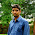I want synchronization generator not alternator

4.Best ans and,BT sir explain abt generator.

5.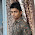Good ans sir...

6.Good ans sir...

7.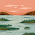8.good explanation ....